# Angles

The candidates preparing for the CAT should be well versed with the quantitative topics to score well in the exam. One such important topic is the “Angles” from which multiple questions might be asked in the exam. So, here is a brief lesson on the “Angles” topic to help the candidates prepare the topic properly.

### Angles Definition:

An angle consists of two rays that share the same endpoint. The point where the rays intersect is called the vertex of the angle. The two rays are called the sides of the angle.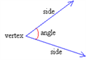#### Naming an Angle

You can name an angle by specifying three points: two on the rays and one at the vertex. The angle below may be specified as angle ABC or ABC. The vertex point is always given in the middle.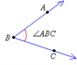#### Angles Around a Point

The sum of angles around a point will always be 360 degrees.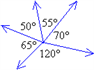In the diagram above, the sum of the angles is 70° + 55° + 50° + 65° + 120° = 360°.

### Types Of Angles:

• #### Right Angles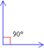• #### Acute Angles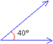• #### Obtuse Angles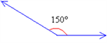• #### Straight Angles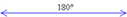• #### Complementary Angles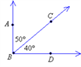ABC is the complement of CBD as 50°+40°=90°

• #### Supplementary Angles

Two angles are called supplementary angles if the sum of their degree measurements equals 180 degrees. One of the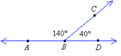supplementary angles are said to be the supplement of the other.

ABC is the supplement of CBD as = 140°+40°= 180 ° Vertically opposite Angles Two pairs of angles are formed by two intersecting lines. Vertical angles are opposite angles in such an intersection. Vertically opposite angles are equal to each other.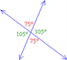• #### Interior Alternate Angles

When a line intersects a pair of parallel lines interior alternate angles are formed. Interior Alternate angles are equal to each other.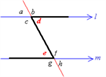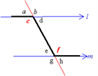In the above diagrams, d and e are alternate interior angles. Similarly, c and f are also alternate interior angles.

• #### Exterior Alternate Angles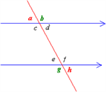a and h are alternate exterior angles and they are equal to one another.

b and g are alternate exterior angles and they are equal to one another.

• #### Corresponding Angles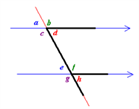In the above diagram, d and h are corresponding angles. Other corresponding angles: b and f; c and g; a and e.

A right angle is an angle measuring 90 degrees. Two lines or line segments that meet at a right angle are said to be perpendicular.An acute angle is an angle measuring between 0 and 90 degrees.An obtuse angle is an angle measuring between 90 and 180 degrees.A straight angle is a straight line and it measures 180 degrees.Two angles are called complementary angles if the sum of their degree measurements equals 90 degrees. One of the complementary angles is said to be the complement of the other.Exterior Alternate angles are equal to one another.When a line intersects a pair of parallel lines corresponding angles are formed. Corresponding angles are equal to each other.

Get all the important lessons and CAT 2017 notification at Byju’s. Also get the latest CAT syllabus and pattern to strategies a proper preparation plan.